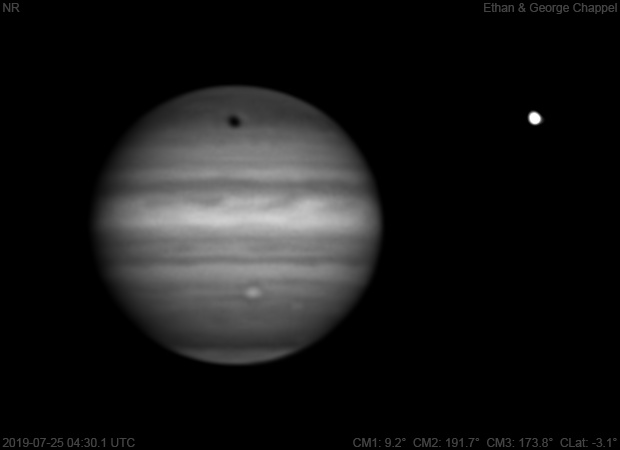# Jupiter 2019-07-25 04:30 UTC

CM1: 9.20°

CM2: 191.70°

CM3: 173.80°

CLat: -3.10°

Description

Less windy conditions allowed us to use the CGEM DX/C14 for the first time in nine days. Seeing was worse than most other nights this summer.

Notes:

• Oval BA is just past the central meridian.
• The STB Ghost, which is pushing against Oval BA from the west, is not visible in methane band.
• The South Polar Red Spot is about to set.
• Ganymede's shadow is crossing the northern hemisphere.

Equipment

ZWO ASI290MM

Celestron EdgeHD 14

Logs
```FireCapture v2.6  Settings
------------------------------------
Observer=Ethan Chappel
Camera=ZWO ASI290MM
Filter=CH4
Profile=Jupiter
Diameter=43.37"
Magnitude=-2.46
CMI=10.8° CMII=193.2° CMIII=175.3°  (during mid of capture)
FocalLength=3650mm (F/13)
Resolution=0.16"
Filename=2019-07-25-0432_3-EC-CH4-Jup.ser
Date=2019_07_25
Start=04_30_19.785
Mid=04_32_19.809
End=04_34_19.833
Start(UT)=04_30_19.785
Mid(UT)=04_32_19.809
End(UT)=04_34_19.833
Duration=240.048s
Date_format=yyyy_MM_dd
Time_format=HH_mm_ss
LT=UT -6h
Frames captured=3693
File type=SER
Binning=no
Bit depth=8bit
Debayer=no
ROI=712x386
ROI(Offset)=0x0
FPS (avg.)=15
Shutter=65.00ms
Gain=400 (66%)
AutoExposure=off
SoftwareGain=10 (off)
Brightness=1 (off)
FPS=100 (off)
AutoGain=off
AutoHisto=75 (off)
Gamma=50 (off)
HighSpeed=off
USBTraffic=40 (off)
Histogramm(min)=0
Histogramm(max)=146
Histogramm=57%
Noise(avg.deviation)=2.16
AutoAlign=false
PreFilter=none
Limit=240 Seconds
Sensor temperature=29.0°C
Focuser position=3724
FireCapture v2.6  Settings
------------------------------------
Observer=Ethan Chappel
Camera=ZWO ASI290MM
Filter=CH4
Profile=Jupiter
Diameter=43.37"
Magnitude=-2.46
CMI=8.1° CMII=190.6° CMIII=172.7°  (during mid of capture)
FocalLength=3650mm (F/13)
Resolution=0.16"
Filename=2019-07-25-0428_0-EC-CH4-Jup.ser
Date=2019_07_25
Start=04_26_00.501
Mid=04_28_00.511
End=04_30_00.521
Start(UT)=04_26_00.501
Mid(UT)=04_28_00.511
End(UT)=04_30_00.521
Duration=240.020s
Date_format=yyyy_MM_dd
Time_format=HH_mm_ss
LT=UT -6h
Frames captured=3693
File type=SER
Binning=no
Bit depth=8bit
Debayer=no
ROI=712x386
ROI(Offset)=0x0
FPS (avg.)=15
Shutter=65.00ms
Gain=400 (66%)
AutoExposure=off
SoftwareGain=10 (off)
Brightness=1 (off)
FPS=100 (off)
AutoGain=off
AutoHisto=75 (off)
Gamma=50 (off)
HighSpeed=off
USBTraffic=40 (off)
Histogramm(min)=0
Histogramm(max)=140
Histogramm=54%
Noise(avg.deviation)=2.16
AutoAlign=false
PreFilter=none
Limit=240 Seconds
Sensor temperature=29.2°C
Focuser position=3724
```